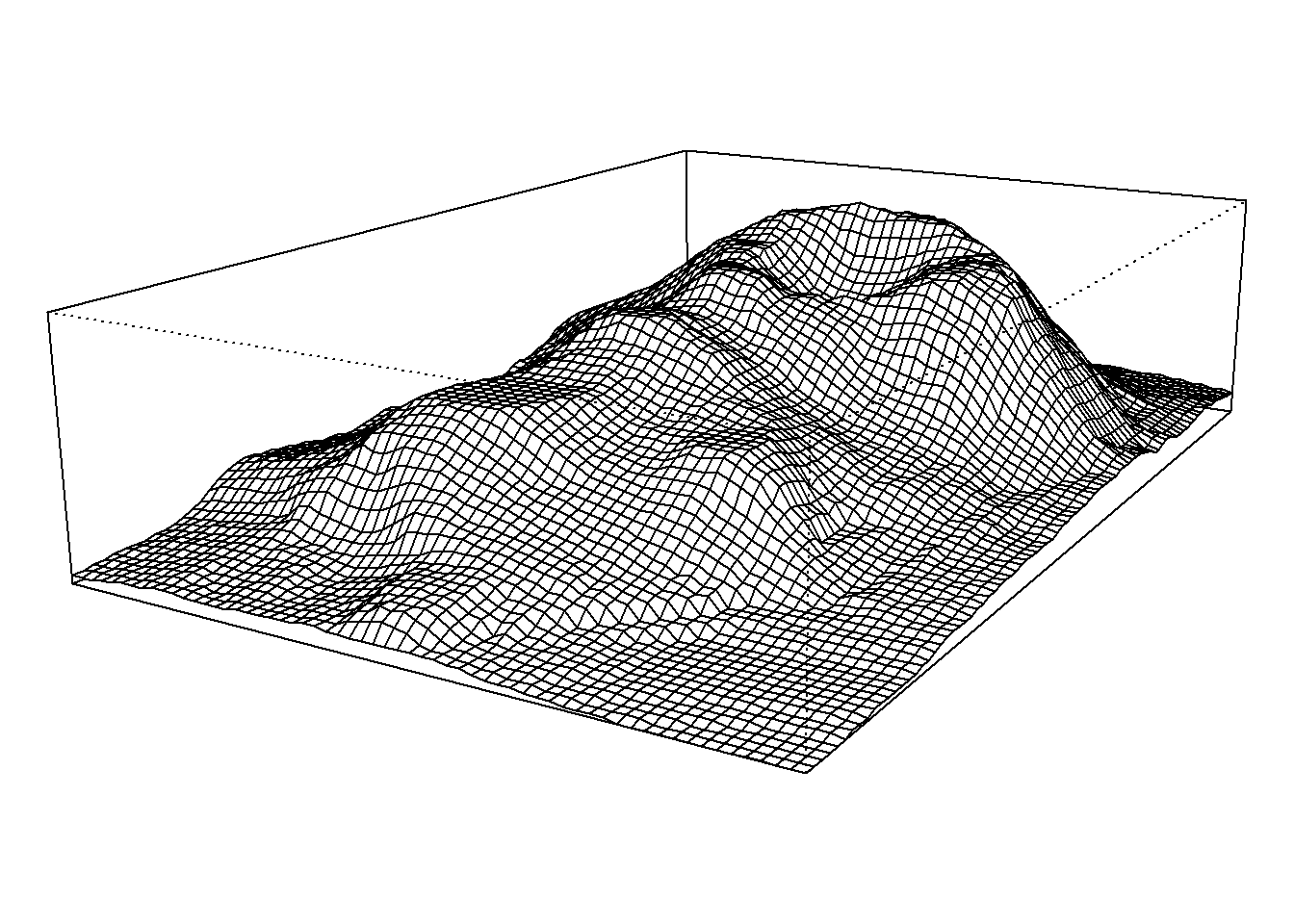# 前言

 persp(x, y, z, theta = 120, phi = 15, scale = FALSE,
axes = FALSE)$\int_0^\infty e^{-x^2} dx=\frac{\sqrt{\pi}}{2}$